# Closure in JavaScript

Most of the JavaScript Developers use closure consciously or unconsciously. Even if they do unconsciously it works fine in most of the cases. But knowing closure will provide better control over the code when using them. And another reason for learning closure is that it is the most frequently asked question in the interview for the JavaScript developers.

Prerequisite:: Variable Scope in JavaScript

Let’s see and understand closure through an example.
Example 1:

 `// Explanation of closure ` `/* 1 */`        `function` `foo() ` `/* 2 */`         `{ ` `/* 3 */`             `var` `b = 1; ` `/* 4 */`             `function` `inner(){ ` `/* 5 */`                 `return` `b; ` `/* 6 */`             `} ` `/* 7 */`             `return` `inner; ` `/* 8 */`         `} ` `/* 9 */`         `var` `get_func_inner = foo();          ` ` `  `/* 10 */`         `console.log(get_func_inner()); ` `/* 11 */`         `console.log(get_func_inner()); ` `/* 12 */`         `console.log(get_func_inner()); `

Explanation:Interesting thing to note here is from line number 9 to line number 12 . At line number 9 we are done with the execution of function foo() and the entire body of function inner() is returned and stored in var get_func_inner, due to the line 7 return inner.
[The return statement does not execute the inner function – function is executed only when followed by () , but rather the return statement returns the reference to the function as a function in JavaScript is also an object.]

We can access the variable b which is defined in function foo() through function inner() as the later preserves the scope chain of enclosing function at the time of execution of enclosing function i.e. the inner function knows the value of b through it’s scope chain.
This is closure in action that is inner function can have access to the outer function variables as well as all the global variables.
Output of the above code: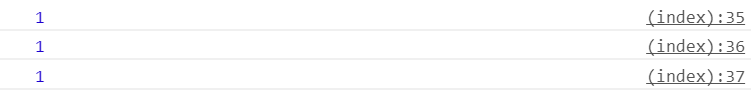In order to see the variable and function bound within closure we can write as:

 `/* 13 */`         `console.dir(get_func_inner); `

Output: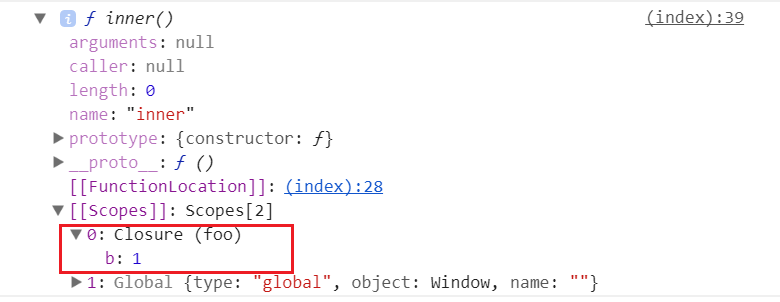As we can see the variables within the closure in the scope section.

Definition of Closure:

In programming languages, closures (also lexical closures or function closures) are techniques for implementing lexically scoped name binding in languages with first-class functions. Operationally, a closure is a record storing a function[a] together with an environment: a mapping associating each free variable of the function (variables that are used locally, but defined in an enclosing scope) with the value or reference to which the name was bound when the closure was created.[b]
-Wikipedia

or

In other words, closure is created when a child function keep the environment of the parent scope even after the parent function has already executed

Now lets look at the another example.
Example 2:

 `function` `foo(outer_arg) { ` ` `  `    ``function` `inner(inner_arg) { ` `        ``return` `outer_arg + inner_arg; ` `    ``} ` `    ``return` `inner; ` `} ` `var` `get_func_inner = foo(5); ` ` `  `console.log(get_func_inner(4)); ` `console.log(get_func_inner(3)); `

Explanation: In the above example we used a parameter function rather than a default one. Note even when we are done with the execution of foo(5) we can access the outer_arg variable from the inner function. And on execution of inner function produce the summation of outer_arg and inner_arg as desired.
Output: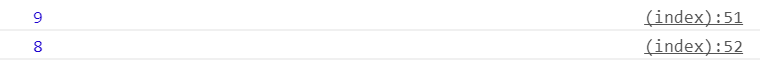Now let’s see an example of closure within a loop.
In this example we would to store a anonymous function at every index of an array.
Example 3:

 `// Outer function ` `function` `outer()  ` `{ ` `    ``var` `arr = []; ` `    ``var` `i; ` `    ``for` `(i = 0; i < 4; i++)  ` `    ``{ ` `        ``// storing anonymus function ` `        ``arr[i] = ``function` `() { ``return` `i; } ` `    ``} ` ` `  `    ``// returning the array. ` `    ``return` `arr; ` `} ` ` `  `var` `get_arr = outer(); ` ` `  `console.log(get_arr()); ` `console.log(get_arr()); ` `console.log(get_arr()); ` `console.log(get_arr()); `

Output: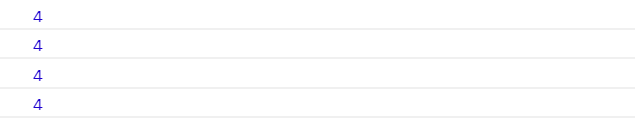Explanation: Did you guess the right answer? In the above code we have created four closure which point to the variable i which is local variable to the function outer. Closure don’t remember the value of the variable it only points to the variable or stores the reference of the variable and hence, returns the current value. In the above code when we try to update the value of it gets reflected to all because the closure stores the reference.
Lets see an correct way to write the above code so as to get different values of i at different index.
Example 4:

 `// Outer function ` `function` `outer()  ` `{ ` `    ``function` `create_Closure(val)  ` `    ``{ ` `        ``return` `function``()  ` `        ``{ ` `            ``return` `val; ` `        ``} ` `    ``} ` `    ``var` `arr = []; ` `    ``var` `i; ` `    ``for` `(i = 0; i < 4; i++)  ` `    ``{ ` `        ``arr[i] = create_Closure(i); ` `    ``} ` `    ``return` `arr; ` `} ` `var` `get_arr = outer(); ` `console.log(get_arr()); ` `console.log(get_arr()); ` `console.log(get_arr()); ` `console.log(get_arr()); `

Output: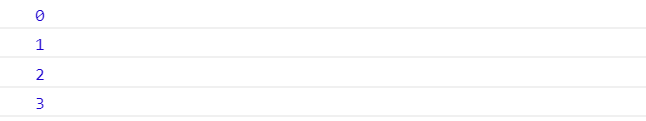Explanation: In the above code we are updating the argument of the function create_Closure with every call. Hence, we get different values of i at different index.

Note : It may be slightly difficult to get the concept of closure at once but try experimenting with closure in different scenarios like for creating getter/setter, callbacks and so on.
Reference:
“Object Oriented JavaScript” by Stoyan Stefanov
This article is contributed by Sumit Ghosh. If you like GeeksforGeeks and would like to contribute, you can also write an article using contribute.geeksforgeeks.org or mail your article to contribute@geeksforgeeks.org. See your article appearing on the GeeksforGeeks main page and help other Geeks.My Personal Notes arrow_drop_up

Article Tags :

11

Please write to us at contribute@geeksforgeeks.org to report any issue with the above content.